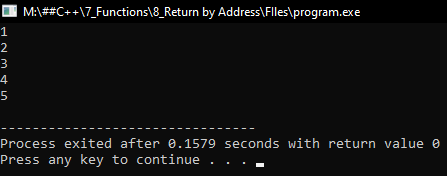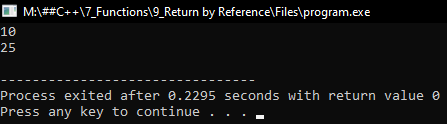# Function Return by Address and Reference in C++

## Function Return by Address and Reference in C++:

In this article, I am going to discuss Function Return by Address and Reference in C++ Language with examples. Please read our previous article, where we discussed Parameter Pass by References in C++ with examples.

##### Function Return by Address in C++:

Here, we will discuss about return type of function that is return by address. A function can also return addresses. Function can take parameters as addresses and when they do so, they are called as Parameter Pass by address and also a function in C++ can return an address also. Let us see some examples of function which returns an address.

int* fun(int size){
int *p = new int[size];
for(int i = 0; i < size; i++){
p[i] = i+1;
}
return p;
}
int main(){
int *ptr = fun(5);
}

Here we have written a function ‘fun’ and it takes one parameter ‘size’. Next, we have taken one pointer and created an array of that size. So, where the memory is allocated? The ‘new’ keyword is used here so the memory is created inside the heap memory. So, this is creating an array inside the heap memory and ‘p’ is a pointer to that memory.

Next, we have a loop here that is taking us through all these elements of Array ‘p’ and assigning every element of array to ‘i+1’. This will fill the elements with natural numbers starting from 1 to 5. Now ‘return p’. What is ‘p’? ‘p’ is a pointer of type Integer. This function is useful for creating an array and initializing all the elements from 1 through whatever size we have mentioned. And then it will return a pointer to that array.

Next, we have called this ‘fun’ function inside the main function. And store the result of ‘fun’ in the pointer ‘ptr’. As the ‘fun’ function is returning a pointer so we have taken an ‘int’ pointer to store the results. We have given ‘5’ as size, so spaces for 5 ‘int’ values will be created inside the heap memory. One important point is function is returning the pointer of the ‘int’ type, so we have taken a function of type ‘int *’.

This function has created some memory in the heap and it has not been deleted. So, the heap memory will be as it is and it is handing over that address to the main function. So ‘ptr’ is a pointer to that one. So, if you have any work related to heap memory then those functions can return the address of that heap memory and it will be useful for the calling function and the main can access that memory. Now let us write a full program.

##### Function Return by Address Program in C++:
```#include <iostream>
using namespace std;

int* fun (int size)
{
int *p = new int[size];
for (int i = 0; i < size; i++)
{
p[i] = i + 1;
}
return p;
}

int main()
{
int *ptr = fun (5);
for (int j = 0; j < 5; j++)
{
cout << ptr[j] << endl;
}
}
```
###### Output:• A function can return the address of the memory
• It should not return the address of local variables, which will be disposed of after the function ends
• It can return the address of memory allocated in the heap

#### Function Return by Reference in C++

Now, we will look at function return by reference. Let us understand this with an example. Please have a look at the below piece of code.

int& fun(int &a){
cout << a << endl;
return a;
}
int main(){
int x = 10;
fun(x) = 25;
cout << x << endl;
}

Here, inside the main function, we have declared a variable x and initialized this variable with a value of 10. Next, we are calling the function ‘fun’ by passing x. So, this function is taking ‘a’ integer variable which is a reference to variable ‘x’.

We know one thing when the function returns something that returns value, we can take it in some variable i.e. ‘int y = fun(x)’. Yes, return result we can take it in some variable, so function always comes on the right side of the assignment. We don’t write them on the left-hand side.

Here ‘a’ is not a separate variable, it’s a reference to ‘x’. So, this is called by reference. ‘fun’ will display ‘a’, so it will display 10 then return ‘a’. So, the return type of function is ‘int &’. It will not return the value of ‘’a, it will return the reference, reference of ‘x’ so when it returns here this function ‘fun(x)’ becomes nothing but ‘x’.

So, this whole thing ‘fun(x)’ becomes X only. We can write here ‘fun(x) = 25’. So now you can see an amazing thing here that our function is written on the left-hand side. And our function is acting as a reference of this variable ‘x’.

This is one of the amazing features of C++ on the left-hand side of the assignment which is the L value. You can make the function as L value. Mostly we make the function as R values but you can make it as L value also with the help of written by reference. Now let us write the complete program for call by reference.

##### Function Return by Reference Program in C++:
```#include <iostream>
using namespace std;
int& fun(int &a)
{
cout << a << endl;
return a;
}

int main()
{
int x = 10;
fun(x) = 25;
cout << x << endl;
}
```
###### Output:##### Return by Reference
• A function cal return reference.
• It should not return the reference of its local variables.
• It can return formal parameters if they are references.